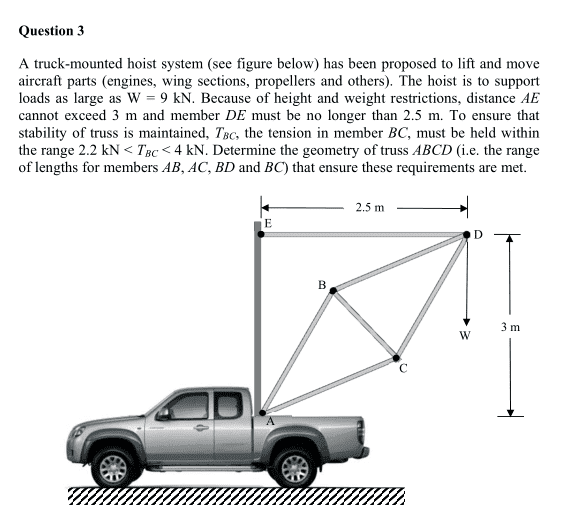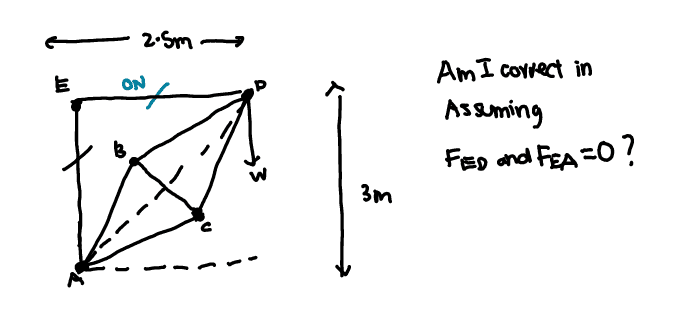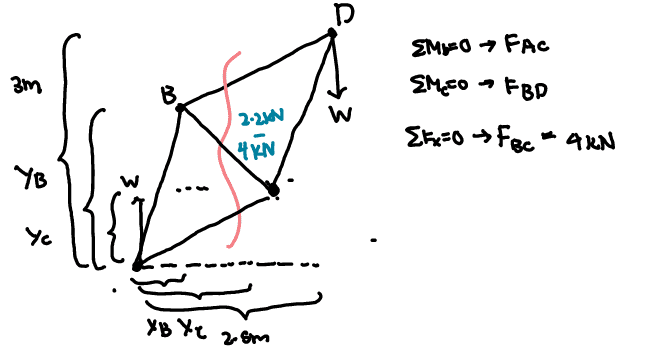# Simple Statics Question: Truss Structure

cloudsync

## Homework Statement## Homework Equations

∑M = 0, ∑Fx = 0, ∑FY = 0

## The Attempt at a SolutionIf so,If so, I will be using the method of sections to cut along the red line as indicated in the picture.

This gives me 3 equations of equilibrium as shown above. However, before solving these equations i first need to check whether my equations/diagrams are contain all the forces necessary.

I know that there is a support reaction in the Y direction of magnitude W at point A.
However, where do I put the support reaction in the x direction? From what I understand there needs to be a support reaction in the X direction as there is a moment generated from the W down and the W up.